Einstein's Theory of Relativity

## Classical Mechanics

by   Paul Marmet
Chapter Eight
The Doppler Effect.
8.1 - Fundamental Principles of the Doppler Effect.
In chapter two, we considered the special case of zero Doppler effect. This means that the source was moving in a direction perpendicular to the direction of propagation of light. The change of frequency due to the Doppler effect was zero because the radial velocity between the source and the detector was equal to zero. When there is a relative radial velocity between the source and the detector, the Doppler effect must be taken into account. Unfortunately, this phenomenon does not seem to be completely well understood in physics.
There have been many discussions about the question of the conservation of energy in the Doppler effect. For example, Weiss and Baez wrote an article  entitled: "Is Energy Conserved in General Relativity?"
They consider the case of the cosmic radiation that has been redshifted over billions of years. "Each photon gets redder and redder. What happens to this energy?" They report that:"... the energy is simply lost".
Such an answer is not acceptable since we believe in mass-energy conservation. We do not believe that any kind of energy can ever be lost whatever the circumstances are. If this were possible, energy would be created from nothing when an emitter moves toward an observer because of the Doppler effect.
Of course, an increasing radial velocity necessarily produces a reddening but one sees that a reddening is not a proof of a Doppler effect since it can be produced by other ways. It has been shown  that the reddening of the cosmic radiation can be better explained by a different phenomenon in which mass-energy is conserved. The reddening results from the energy lost following numerous interactions of photons on interstellar gases during billions of years. In that case, the residual energy is scattered elsewhere so that there is no difficulty to be compatible with the principle of mass-energy conservation.

8.2 - Mass-Energy Conservation in the Context of the Doppler Effect.
Doppler reddening is a real phenomenon which can occur in some cases and which is always compatible with mass-energy conservation. For example, let us consider the case of a hydrogen atom excited to 10.2 eV (the Lyman state) moving away from a stationary source. If the hydrogen atom moves at half the velocity of light, the theory of the Doppler effect (using the wave property of light) teaches us that we will receive only half of the frequency of the excited state. This means that the photon received from the moving particle will have only half the energy of excitation. The question is: Where does the difference of energy (5.1 eV) go? It has been claimed in several papers that the energy is missing.
The demonstration using the change of frequency of a wave due to the relative velocity does not take into account all the energy available in the experiment. Let us calculate the Doppler effect without using waves but using only the principle of mass-energy conservation.

8.3 - The Doppler Effect without Using Waves.
Let us consider a mass mo (at rest) moving away at velocity V with respect to an observer at rest. Let us assume that the mass is a hydrogen atom. This moving atom has a total energy of: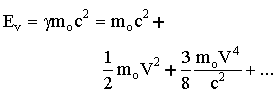8.1
Let us consider the case when that hydrogen atom is excited at the Lyman a atomic state with an energy hno of 10.2 eV. The total energy (potential plus kinetic) of that excited atom (neglecting the higher order terms) is: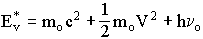8.2
Let us use the moving frame of the particle from which the photon is emitted. To be detected in the rest frame, the photon must be emitted backward (-x axis) from the moving atom, in the direction of the rest frame where the observer is located. When the photon is emitted, the atom gets a recoil in the forward (+x axis) direction giving it an increase of velocity Dv. Of course, the total change of momentum DP of the moving system (photon plus atom) is zero. At the moment of emission, considering the photon's momentum, we have: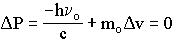8.3
or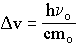8.4
With respect to the rest frame, the velocity of the hydrogen atom was V before the emission of the photon. After the emission of the photon, the final velocity Vf of the atom with respect to the rest frame becomes:
 Vf = V + Dv 8.5
Equation 8.4 in 8.5 gives: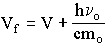8.6
The total (mass plus kinetic) energy of the de-excited hydrogen atom after the emission of the photons is (neglecting the higher order terms):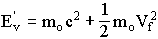8.7
Using equation 8.6 gives:8.8
The change of kinetic energy of the hydrogen atom due to the recoil of the photon is:8.9
From equations 8.8 and 8.1, neglecting the second order, we have:8.1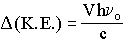8.11
Equation 8.11 gives the increase of kinetic energy of the atom due to its recoil. According to the mass-energy conservation principle, the increase of kinetic energy of the atom must come from the photon energy. Since the excitation energy initially available was hno, and since equation 8.11 gives the energy transferred to the atom (as kinetic energy), the residual photon energy hnf is:8.12
which is:8.13
Equation 8.13 is exactly identical to the Doppler equation.
We have demonstrated the Doppler equation using no wave model but only mass-energy conservation. The energy apparently lost in the Doppler phenomenon is simply transferred as kinetic energy to the emitting atom whose velocity has increased due to the recoil momentum. It is also important to notice that the amount of kinetic energy lost in equation 8.11 is independent of the mass of the particle.
The above demonstration solves the problem discussed by Weiss and Baez and others. We conclude that the energy redshifted by the Doppler mechanism is not lost. It is simply transmitted as kinetic energy to the emitting atom due to recoil at the moment of emission. We must notice that this explanation has nothing to do with relativity.

8.4 - References.
 http://www-hpcc.astro.washington.edu/mirrors/physicsfaq/energy_gr.html
 P. Marmet, A New Non-Doppler Redshift,    Physics Essays, 1, 1, P. 24-32, 1988.

8.5 - Symbols and Variables.

 EV energy of a mass mo moving at velocity V E*V energy of a mass mo moving at velocity V and excited to 10.2 eV E'V energy of a mass mo moving at velocity V after losing its energy of excitation no frequency corresponding to the excitation energy nf frequency emitted by the atom
<><><><><><><><><><><><>
Chapter7   Contents      Chapter 9